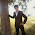Basic Salary C Program Flow Char

This C program to calculate the Basic Salary of a Employee with Flow Chart. This is a part of Mumbai University MCA Colleges C Program MCA Sem 1

A program to read basic from the user and calculate net and gross salary with flow chart.

Where

Da=41% of basic salary,
Hra=30% of basic salary,
Pf=12% of basic salary,
It=300,
Cca=800.

#include<stdio.h>
#include<conio.h>

void main()
{
/*variable declaration*/
float basic_salary,da,hra,cca,pf,it,gross_salary,net_salary;
clrscr();
printf("\nEnter the basic salary of an employee: ");
scanf("%f",&basic_salary);

/*calculation*/
da = basic_salary*41/100;
hra =basic_salary *30/100;
cca = 800;
pf = basic_salary*12/100;
it = 300;
gross_salary=basic_salary+hra+cca+da;
net_salary=gross_salary-pf-it;
printf("\nGross Salary is %.2f",gross_salary);
printf("\nNet Salary is %.2f",net_salary);
getch();
}

Flow Chart

Hope this Program is useful to you in some sense or other. Keep on following this blog for more Mumbai University MCA College Programs. Happy Programming and Studying.

1 comment:

1.